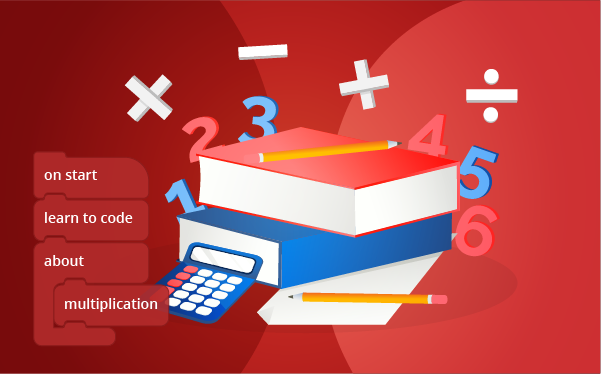Elementary School course

# Math 101

• BEGINNER

1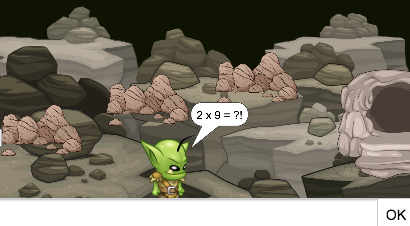Multiplication Escape
Create a project that demonstrates your knowledge of multiplication.

2Identifying Shapes
Create a project that demonstrates your knowledge of shapes.

3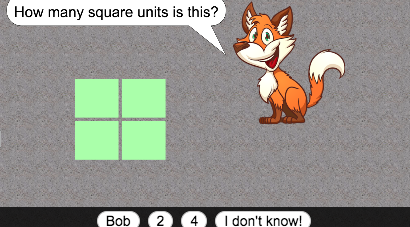Finding Area
Create a project that demonstrates your knowledge of finding the area in shapes.

4Multiplication
Create a project that tests your multiplication knowledge.

5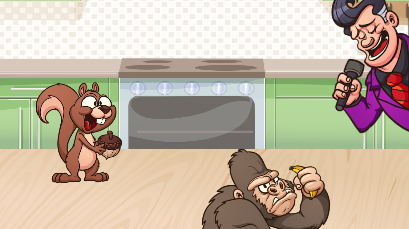Division
Create a project that tests your division knowledge.

6Ninja Math Tables
Create a project that tests your knowledge of math tables.

7Rounding Roundup
Create a project that tests your knowledge of rounding numbers.

8Division Duel
Create a game that is all about division.

9Fraction Number Line
Create a project that demonstrates fraction principles.

10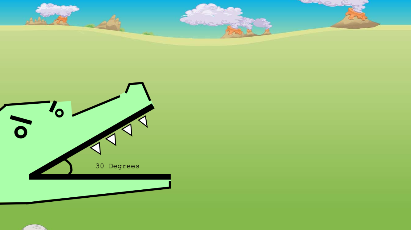Angles
Create a project that demonstrates your knowledge of angles.

11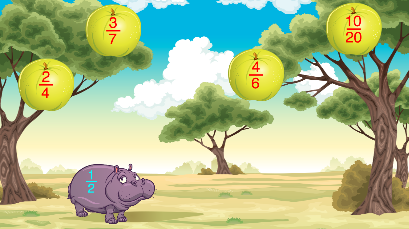Equal Fractions
Create a project that demonstrates your knowledge of equal fractions.

12Order of Operations
Create a project that demonstrates your knowledge of order of operations.

13Symmetry
Create a project that demonstrates your knowledge of symmetry.

14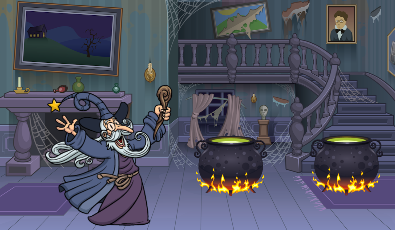Create a project that demonstrates your knowledge of fractions.

15Parallel/Perpendicular
Create a project that demonstrates your knowledge of parallel and perpendicular lines.

16Making Change
Create a project that demonstrates your knowledge of making change.

17Alien Change
Create a project that demonstrates your knowledge of making change.

18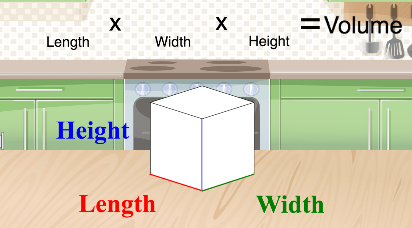Volume
Create a project that demonstrates your knowledge of finding the volume of shapes.

1920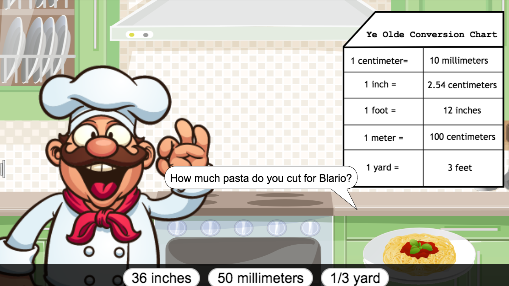Unit Conversion
Create a project that demonstrates your knowledge of unit conversions.

21Long Division
Create a project that demonstrates your knowledge of long division.

22Telling Time
Create a project that demonstrates your knowledge of telling time.

23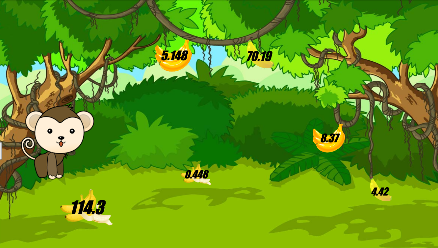Decimal Math Quiz
Create a decimal math quiz.

24Decimal Places
Create a project that demonstrates your knowledge of decimal places.